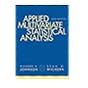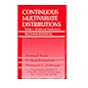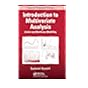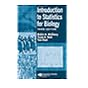Normal view

# Introduction to multivariate analysis : linear and nonlinear modeling / Sadanori Konishi.

Material type:TextLanguage: English Publication details: 2014. Description: xxv, 312 p. : illus. ; 24 cmISBN: 9781466567283 (hardback); 1466567287Subject(s): Multivariate analysisDDC classification: 519.535 LOC classification: QA278 | .K597 2014Online resources: WorldCat details | E-book Fulltext
Contents:
TOC Introduction Regression Modeling Classification and Discrimination Dimension Reduction Clustering Linear Regression Models Relationship between Two Variables Relationships Involving Multiple Variables Regularization Nonlinear Regression Models Modeling Phenomena Modeling by Basis Functions Basis Expansions Regularization Logistic Regression Models Risk Prediction Models Multiple Risk Factor Models Nonlinear Logistic Regression Models Model Evaluation and Selection Criteria Based on Prediction Errors Information Criteria Bayesian Model Evaluation Criterion Discriminant Analysis Fisher's Linear Discriminant Analysis Classification Based on Mahalanobis Distance Variable Selection Canonical Discriminant Analysis Bayesian Classification Bayes' Theorem Classification with Gaussian Distributions Logistic Regression for Classification Support Vector Machines Separating Hyperplane Linearly Nonseparable Case From Linear to Nonlinear Principal Component Analysis Principal Components Image Compression and Decompression Singular Value Decomposition Kernel Principal Component Analysis Clustering Hierarchical Clustering Nonhierarchical Clustering Mixture Models for Clustering Appendix A: Bootstrap Methods Appendix B: Lagrange Multipliers Appendix C: EM Algorithm Bibliography Index
Summary: "Multivariate techniques are used to analyze data that arise from more than one variable in which there are relationships between the variables. Mainly based on the linearity of observed variables, these techniques are useful for extracting information and patterns from multivariate data as well as for the understanding the structure of random phenomena. This book describes the concepts of linear and nonlinear
Tags from this library: No tags from this library for this title.
Star ratingsAverage rating: 0.0 (0 votes)
Holdings
Item type Current library Collection Call number Copy number Status Date due Barcode Item holdsE-Book
E-book
Non-fiction 519.535 KOI 2014 (Browse shelf(Opens below)) Not for loanText
Reserve Section
Non-fiction 519.535 KOI 2014 (Browse shelf(Opens below)) C-1 Not For Loan 27387Text
Circulation Section
Non-fiction 519.535 KOI 2014 (Browse shelf(Opens below)) C-2 Available 27388Text
Circulation Section
Non-fiction 519.535 KOI 2014 (Browse shelf(Opens below)) C-3 Available 27389Text
Circulation Section
Non-fiction 519.535 KOI 2014 (Browse shelf(Opens below)) C-4 Available 27390
Total holds: 0
##### Browsing EWU Library shelves, Shelving location: E-book Close shelf browser (Hides shelf browser)519.535 IZM 2008 Modern multivariate statistical techniques : 519.535 JOA 2007 Applied multivariate statistical analysis / 519.535 KOC 2000 Continuous multivariate distributions / 519.535 KOI 2014 Introduction to multivariate analysis : 519.535 MCI 2007 Introduction to statistics for biology / 519.535 NEI 2006 An introduction to copulas / 519.535 PES 2010 A SAS/IML companion for linear models /

Includes bibliographical references (pages 299-307) and index.

TOC Introduction Regression Modeling Classification and Discrimination Dimension Reduction Clustering Linear Regression Models Relationship between Two Variables Relationships Involving Multiple Variables Regularization Nonlinear Regression Models Modeling Phenomena Modeling by Basis Functions Basis Expansions Regularization Logistic Regression Models Risk Prediction Models Multiple Risk Factor Models Nonlinear Logistic Regression Models Model Evaluation and Selection Criteria Based on Prediction Errors Information Criteria Bayesian Model Evaluation Criterion Discriminant Analysis Fisher's Linear Discriminant Analysis Classification Based on Mahalanobis Distance Variable Selection Canonical Discriminant Analysis Bayesian Classification Bayes' Theorem Classification with Gaussian Distributions Logistic Regression for Classification Support Vector Machines Separating Hyperplane Linearly Nonseparable Case From Linear to Nonlinear Principal Component Analysis Principal Components Image Compression and Decompression Singular Value Decomposition Kernel Principal Component Analysis Clustering Hierarchical Clustering Nonhierarchical Clustering Mixture Models for Clustering Appendix A: Bootstrap Methods Appendix B: Lagrange Multipliers Appendix C: EM Algorithm Bibliography Index

"Multivariate techniques are used to analyze data that arise from more than one variable in which there are relationships between the variables. Mainly based on the linearity of observed variables, these techniques are useful for extracting information and patterns from multivariate data as well as for the understanding the structure of random phenomena. This book describes the concepts of linear and nonlinear

AS

Sagar Shahanawaz

There are no comments on this title.